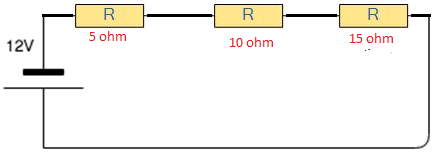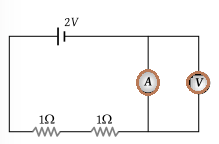## Physics MCQ on Kirchhoff’s Law for NEET, JEE, Medical and Engineering Exam 2021

MCQ on Kirchhoffs Law: Previous trends reveal that NEET/JEE aspirants find Physics as the most challenging section amongst the other sections. But most of them fail to decipher the reason behind this. This particularly can be as the candidates taking up this examination are predominantly medical aspirants with their subject of interest being Biology. Also, sections like Physics are more on theories, laws, numerical as opposed to Biology which is more of fact-based, life sciences and comprising of substantial explanations.In this post we are providing you MCQ on Kirchhoffs Law, which will be beneficial for you in upcoming NEET, AIIMS, JEE, WBJEE, Medical and Engineering Exams.

## MCQ on Kirchhoffs Law

Q1. Kirchhoff’s Current Law is based on ________.
a) The charge can be accumulated at the node
b) Charge cannot be accumulated at the node
c) Energy is stored at the node
d) Depending on the circuit charge can be accumulated at the circuit

(b) Charge cannot be accumulated at the node

Q2. What is the relation between currents in the figure below.a) i2 = i1 + i3 + i4 +i5
b) i2 – i1 = i3 – i4 +i5
c) i3 +i4 =i1+i2+i5
d) I1+i5 =i2+i3+i4

(a) i2 = i1 + i3 + i4 +i5

Q3. The terminal potential difference will be greater than its emf when it is ________.
a) In open circuit
b) Being charged
c) Being charged or discharged
d) Being discharged

(b) Being charged

Q4. Calculate the voltage across the 5ohm resistor.a) 12 V
b) 2 V
c) 10 V
d) 0 V

(b) 2 V

Q5. The algebraic sum of voltages around any closed path in a network is equal to ________.
a) Infinity
b) 1
c) 0
d) Negative polarity

(c) 0

Q6. In Kirchhoff’s first law Σi = 0 at the junction is based on the conservation of ________.
a) Energy
b) Charge
c) Momentum
d) Speed

(b) Charge

Q7. Which basic law should be followed to analyse the circuit?
a) Newton’s law
c) Amperes law
d) Kirchoff’s law

(d) Kirchoff’s law

Q8. When the cells are connected in parallel, then ________.
a) The current increases
b) The current decreases
c) The emf increases
d) The emf decreases

(a) The current increases

Q9. Calculate the value of V1 and V2.a) 4V, 6V
b) 5V,6V
c) 6V,7V
d) 7V,8V

(a) 4V, 6V

Q10. In the circuit shown below what will be the reading in the voltmeter.

a)2 Vb) 1V
c) 0.5V
d) Zero

(d) Zero